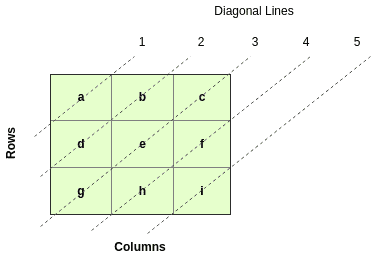## 1. Overview

In this tutorial, we will see how to loop diagonally through a two-dimensional array. The solution that we provide can be used for a square two-dimensional array of any size.

## 2. Two-Dimensional Array

The key in working with elements of an array is knowing how to get a specific element from that array. For a two-dimensional array, we use row and column indices to get elements of an array. For this problem, we’ll use the following diagram to show how to get these elements.Next, we need to understand how many diagonal lines we have in our array, as seen in the diagram. We do this by first getting the length of one dimension of the array and then using that to get the number of diagonal lines (diagonalLines).

We then use the number of diagonal lines to get the mid-point which will help in the search for row and column indices.

In this example, the mid-point is three:

``````int length = twoDArray.length
int diagonalLines = (length + length) - 1
int midPoint = (diagonalLines / 2) + 1``````

## 3. Getting Row and Column Indices

To loop through the whole array, we start looping from 1 until the loop variable is less than or equal to the diagonalLines variable.

``````for (int i = 1; i <= diagonalLines; i++) {
// some operations
}``````

Let’s also introduce the idea of the number of items in a diagonal line, calling it itemsInDiagonal. For example, line 3 in the diagram above has 3 items (g, e, c) and line 4 has 2 (h, f). This variable is incremented by 1 in the loop when loop variable is less or equal to midPoint. It is then decremented by 1 otherwise.

After incrementing or decrementing itemsInDiagonal, we then have a new loop with loop variable j. Variable is incremented from 0 until it is less than itemsInDiagonal.

We then use loop variables and j to get the row and column indices. The logic of this calculation depends on whether loop variable i is greater than midPoint or not. When is greater than midPoint, we also use the length variable to determine the row and column indices:

``````int rowIndex;
int columnIndex;

if (i <= midPoint) {
itemsInDiagonal++;
for (int j = 0; j < itemsInDiagonal; j++) {
rowIndex = (i - j) - 1;
columnIndex = j;
items.append(twoDArray[rowIndex][columnIndex]);
}
} else {
itemsInDiagonal--;
for (int j = 0; j < itemsInDiagonal; j++) {
rowIndex = (length - 1) - j;
columnIndex = (i - length) + j;
items.append(twoDArray[rowIndex][columnIndex]);
}
}``````

## 4. Conclusion

In this tutorial, we have shown how to loop diagonally through a square two-dimensional array using a method that helps in getting row and column indices.

As always, the full source code of the example is available over on GitHub.

### Get started with Spring 5 and Spring Boot 2, through the Learn Spring course:

>> CHECK OUT THE COURSE